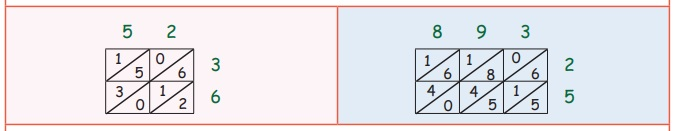Home | | Maths 3rd Std | Lattice multiplication

# Lattice multiplication

Lattice multiplication is helpful while dealing with numbers with more than two digits. We follow the following steps in Lattice multiplication.

Mutiplication of a number with other number can be done in the following ways.

(i) Dot multiplication

(iii) Regrouping

(iv) Standard multiplication algorithm

(v) Lattice multiplication

Lattice multiplication:

Lattice multiplication is helpful while dealing with numbers with more than two digits. We follow the following steps in Lattice multiplication.

(i) 52 × 36 (ii) 893 × 25

Step 1: Write the numbers to be mutiplied as follows.Step 2: Draw diagonals of the square.Step 3: Mulitiply the numbers and write them in the cells as shown belowStep 4 : Find the sum of each diagonal and write as follows.Step 5: Arrange the sum to get answer as follows.Exercise

1. Find the product using standard algorithm:

i) 20 × 2=

ii) 21 × 4 =

iii) 65 × 5 =

iv) 14×3=

v) 26 × 10 =

i) 20 × 2=Product 20 × 2 = 4

ii) 21 × 4 =Product 21 × 4 = 84

iii) 65 × 5 =Product 65 × 5 = 325

iv) 14×3=Product 14 × 3 = 42

v) 26 × 10 =Product 26 × 10 = 260

2. Complete the following table

i) If the cost of one pen is ₹ 5, what will be the cost of 8 pens?

8 × 5 = 40

ii) If there are 7 balls in a bag, how many balls will be there in 4 bags?

4 × 7 = 28

iii) If the cost of one book is ₹ 10, what will be the cost of 7 books?

7 × 10 = 70

iv) If there are 6 buttons in a shirt, how many buttons will be there in 3 shirts?

3 × 6 = 18

3. Find the product of the following numbers using lattice multiplication

i) 22× 25

ii) 34 × 51

iii) 45 × 24

i) 22 × 25ii) 34 × 51iii) 45 × 24